# Assignments

Determine of the following functions (if possible) the vertical and horizontal asymptotes, the intersections with the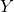- and-axis and sketch the graph.

1.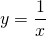Solution

2.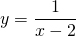Solution

3.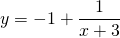Solution

4.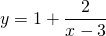Solution

5.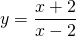Solution

6.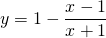Solution

7.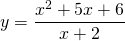Solution

8.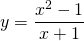Solution

9.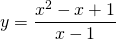Solution

10.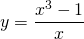Solution

0